# SAT Math Multiple Choice Question 205: Answer and Explanation

### Test Information

Question: 205

10.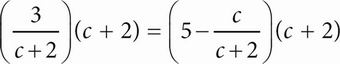In the equation above, what is the value of c ?

• A. -4
• B. -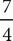• C. -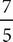• D.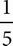B Plugging In would not be straightforward for this problem, given the fractions and negative numbers. A better approach would be to simplify the expressions first and then plug in or solve. Distribute the (c + 2) term to both sides of the equation. On the left side, this will cancel out with the (c + 2) term in the denominator. On the right side, make sure to distribute the (c + 2) to both terms inside the parentheses. The equation becomes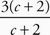= 5(c + 2) -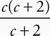or 3 = 5c + 10 - c. Combine the c terms and subtract 10 from both sides to get -7 = 4c. Divide both sides by 4 to find that c = -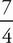. The answer is (B).